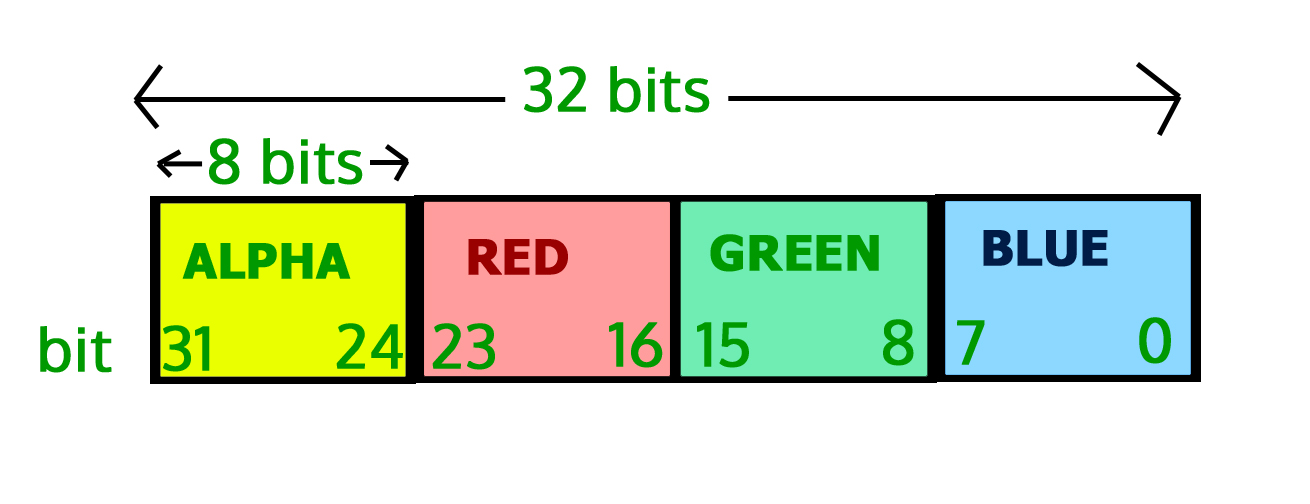GFG App
Open AppBrowser
Continue

# Image Processing In Java – Get and Set Pixels

Prerequisite – Image Processing in Java – Read and Write

In this set, we will learn about the pixels of images, how we can get pixel values of an image and how to set pixel values in an image using Java programming language. Pixels are the smallest unit of an image which consists of four components Alpha (transparency measure), Red, Green, Blue, and in short (ARGB). The value of all the components lies between 0 and 255, both inclusive. Zero means the component is absent, and 255 represents the component is fully present.

Note: Since 28 = 256 and the value of the pixel components lie between 0 and 255, so we need only 8-bits to store the values.

So, the total number of bits required to store the ARGB values is 8*4=32 bits or 4 bytes. As the order signifies, Alpha acquires the leftmost 8 bits. Blue acquires the rightmost 8 bits.

Thus the bit position:

• For the blue component being 7-0,
• For green component being 15-8,
• For the red component being 23-16,
• For alpha component being 31-24,

#### Pictorial Representation of Indexes:## Java

 `// Java program to demonstrate get ` `// and set pixel values of an image ` ` `  `import` `java.awt.image.BufferedImage; ` `import` `java.io.File; ` `import` `java.io.IOException; ` `import` `javax.imageio.ImageIO; ` ` `  `public` `class` `GetSetPixels { ` `    ``public` `static` `void` `main(String args[]) ` `        ``throws` `IOException ` `    ``{ ` `        ``BufferedImage img = ``null``; ` `        ``File f = ``null``; ` ` `  `        ``// read image ` `        ``try` `{ ` `            ``f = ``new` `File( ` `                ``"C:/Users/hp/Desktop/Image Processing in Java/gfg-logo.png"``); ` `            ``img = ImageIO.read(f); ` `        ``} ` `        ``catch` `(IOException e) { ` `            ``System.out.println(e); ` `        ``} ` ` `  `        ``// get image width and height ` `        ``int` `width = img.getWidth(); ` `        ``int` `height = img.getHeight(); ` ` `  `        ``// Since, Inp.jpg is a single pixel image so, we ` `        ``// will not be using the width and height variable ` `        ``// get pixel value (the arguments in the getRGB ` `        ``// method denotes the  coordinates of the image from ` `        ``// which the pixel values need to be extracted) ` `        ``int` `p = img.getRGB(``0``, ``0``); ` ` `  `        ``// We, have seen that the components of pixel occupy ` `        ``// 8 bits. To get the bits we have to first right ` `        ``// shift the 32 bits of the pixels by bit ` `        ``// position(such as 24 in case of alpha) and then ` `        ``// bitwise ADD it with 0xFF. 0xFF is the hexadecimal ` `        ``// representation of the decimal value 255. ` ` `  `        ``// get alpha ` `        ``int` `a = (p >> ``24``) & ``0xff``; ` ` `  `        ``// get red ` `        ``int` `r = (p >> ``16``) & ``0xff``; ` ` `  `        ``// get green ` `        ``int` `g = (p >> ``8``) & ``0xff``; ` ` `  `        ``// get blue ` `        ``int` `a = p & ``0xff``; ` ` `  `        ``// for simplicity we will set the ARGB ` `        ``// value to 255, 100, 150 and 200 respectively. ` ` `  `        ``a = ``255``; ` `        ``r = ``100``; ` `        ``g = ``150``; ` `        ``b = ``200``; ` ` `  `        ``// set the pixel value ` `        ``p = (a << ``24``) | (r << ``16``) | (g << ``8``) | b; ` `        ``img.setRGB(``0``, ``0``, p); ` ` `  `        ``// write image ` `        ``try` `{ ` `            ``f = ``new` `File( ` `                ``"C:/Users/hp/Desktop/Image Processing in Java/GFG.png"``); ` `            ``ImageIO.write(img, ``"png"``, f); ` `        ``} ` `        ``catch` `(IOException e) { ` `            ``System.out.println(e); ` `        ``} ` `    ``} ` `}`

#### Output –

Note: This code will not run on online IDE as it needs an image on disk.